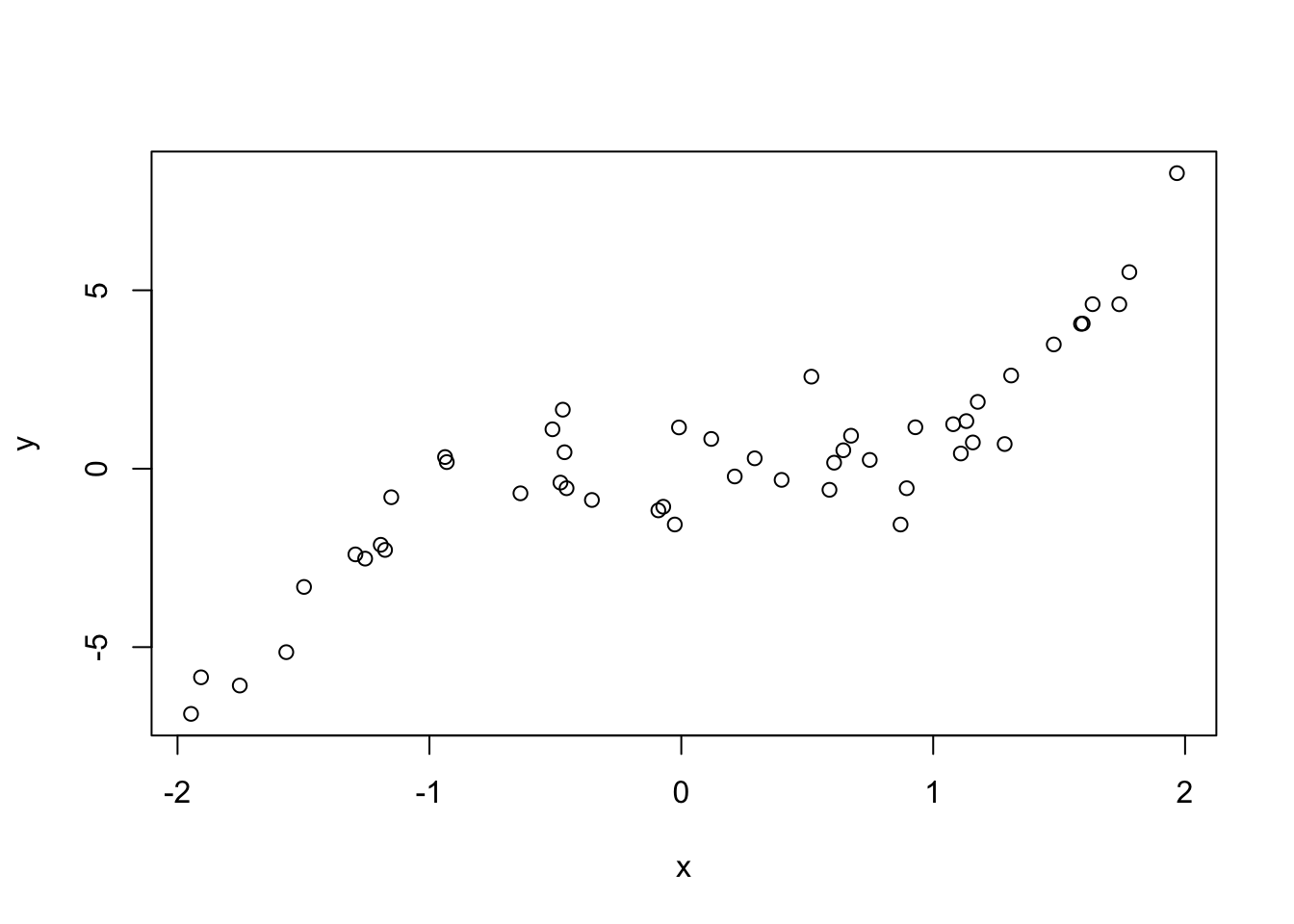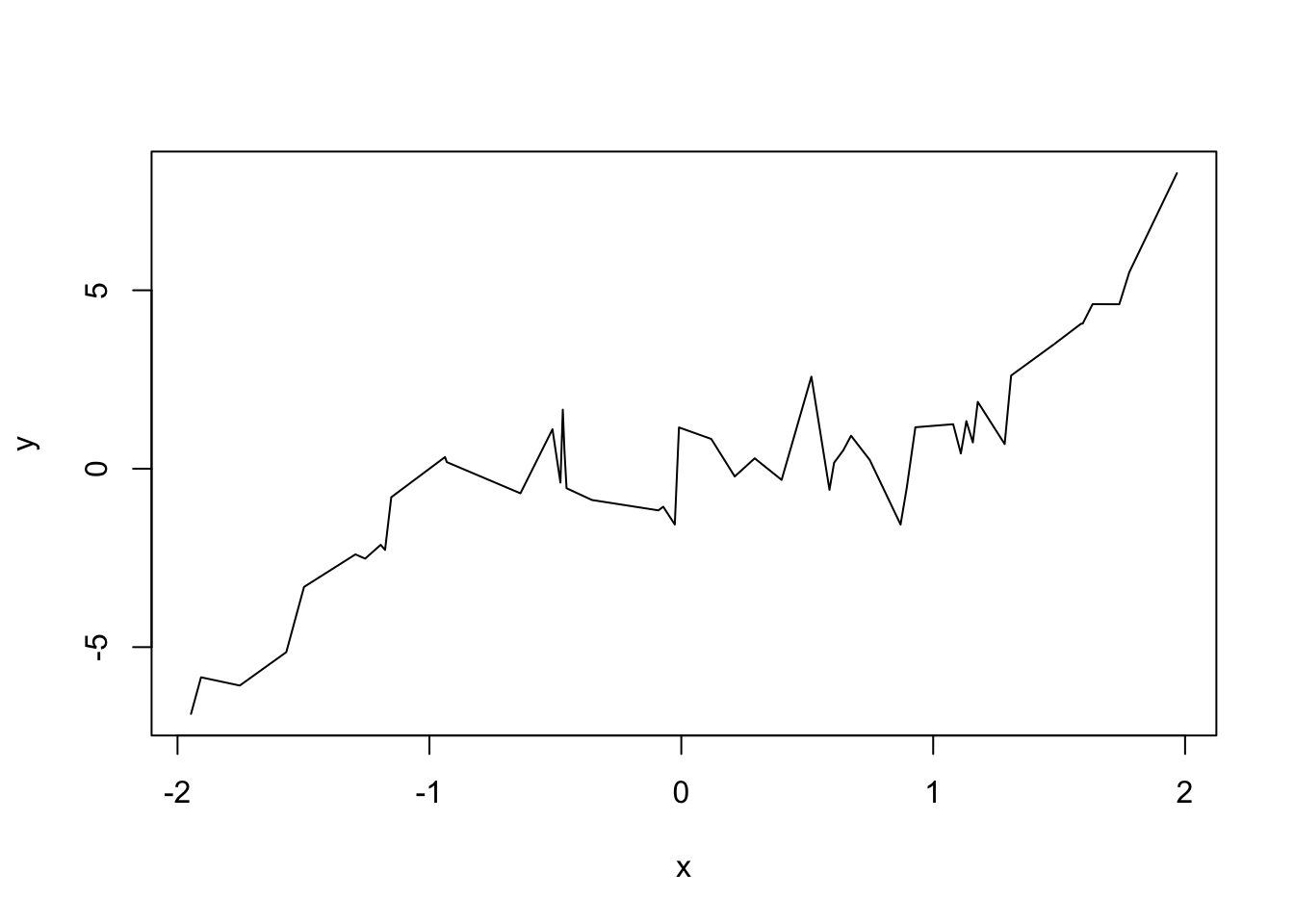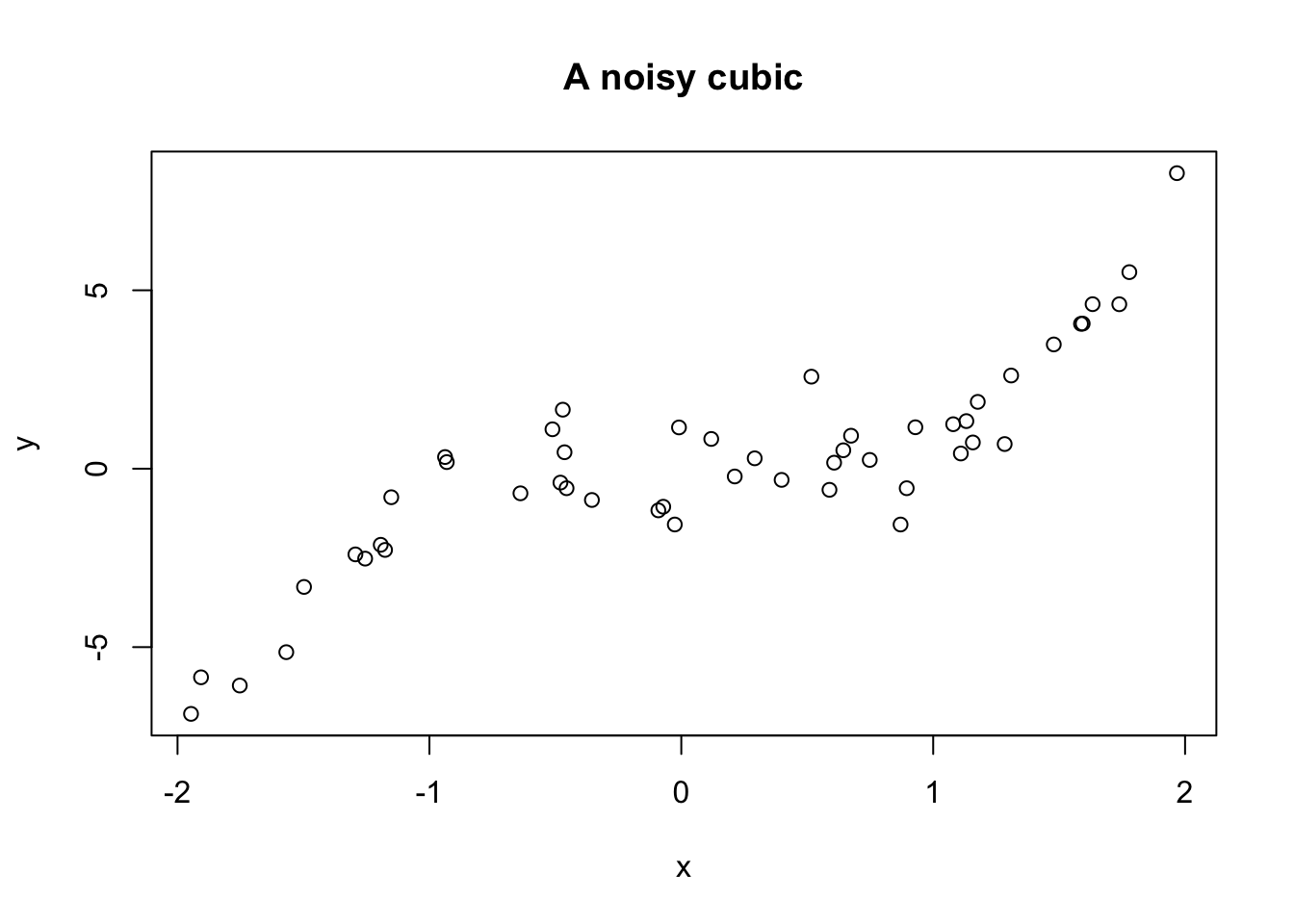# Last week: Text manipulation

• Strings are, simply put, sequences of characters bound together
• Text data occurs frequently “in the wild”, so you should learn how to deal with it!
• `nchar()`, `substr()`: functions for substring extractions and replacements
• `strsplit()`, `paste()`: functions for splitting and combining strings
• Reconstitution: take lines of text, combine into one long string, then split to get the words
• `table()`: function to get word counts, useful way of summarizing text data
• Zipf’s law: word frequency tends to be inversely proportional to (a power of) rank

Plot basics

# Plotting in R

Base R has a set of powerful plotting tools. An overview:

• `plot()`: generic plotting function
• `points()`: add points to an existing plot
• `lines()`, `abline()`: add lines to an existing plot
• `text()`, `legend()`: add text to an existing plot
• `rect()`, `polygon()`: add shapes to an existing plot
• `hist()`, `image()`: histogram and heatmap
• `heat.colors()`, `topo.colors()`, etc: create a color vector
• `density()`: estimate density, which can be plotted
• `contour()`: draw contours, or add to existing plot
• `curve()`: draw a curve, or add to existing plot

The `ggplot2` package also provides very nice (and very different) plotting tools; we won’t cover it in this course (it tends to be the focus in Statistical Graphics, 36-315)

# Scatter plot

To make a scatter plot of one variable versus another, use `plot()`

``````n = 50
set.seed(0)
x = sort(runif(n, min=-2, max=2))
y = x^3 + rnorm(n)
plot(x, y)``````# Plot type

The `type` argument controls the plot type. Default is `p` for points; set it to `l` for lines

``plot(x, y, type="p")````plot(x, y, type="l")``Try also `b` or `o`, for both points and lines

# Labels

The `main` argument controls the title; `xlab` and `ylab` are the x and y labels

``plot(x, y, main="A noisy cubic") # Note the default x and y labels````plot(x, y, main="A noisy cubic", xlab="My x variable", ylab="My y variable")``